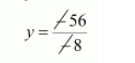# The car hire charges in a city comprise of a fixed charges together with the charge for the distance covered.`
Question:

The car hire charges in a city comprise of a fixed charges together with the charge for the distance covered. For a journey of 12 km, the charge paid is Rs 89 and the journey of 20 km, the charge paid is Rs 145. What will a person have to pay for travelling a distance of 30 km?

Solution:

Let the fixed charges of car be Rs.x per km and the running charges be $R s . y \mathrm{~km} / \mathrm{hr}$

According to the given condition we have

$x+12 y=89 \cdots(i)$

$x+20 y=145 \cdots(i i)$

$x+12 y=89$

$\frac{x+20 y=145}{-8 y=-56}$$y=7$

Putting $y=7$ in equation $(i)$ we get

$x+12 y=89$

$x+12 \times 7=89$

$x+84=89$

$x=89-84$

$x=5$

Therefore, Total charges for travelling distance of $30 \mathrm{~km}$

$=x+30 y$

$=5+30 \times 7$

$=5+210$

$=\operatorname{Rs} 215$

Hence, A person have to pay Rs. 215 for travelling a distance of $30 \mathrm{~km}$.